# Ex 13.9 NCERT Solutions- Surface Areas and Volumes Class 9 Notes | EduRev

## Class 9 : Ex 13.9 NCERT Solutions- Surface Areas and Volumes Class 9 Notes | EduRev

The document Ex 13.9 NCERT Solutions- Surface Areas and Volumes Class 9 Notes | EduRev is a part of the Class 9 Course Class 9 Mathematics by VP Classes.
All you need of Class 9 at this link: Class 9

Question 1. A wooden bookshelf has external dimensions as follows: Height = 110 cm, Depth = 25 cm, Breadth = 85 cm (see the figure). The thickness of the plank is 5 cm everywhere. The external faces are to be polished and the inner faces are to be painted. If the rate of polishing is 20 paise per cm2 and the rate of painting is 10 paise per cm2, find the total expenses required for polishing and painting the surface of the bookshelf.
Solution:
Here, Height = 110 cm
Depth = 25 cm
Thickness of the plank = 5 cm
Cost of polishing
∵ Area to be polished = [(110 x 85)] + [(85 x 25) x 2] + [(110 x 25) x 2] + [(5 x 110) x 2] + [(75 x 5) x 4] cm2
=  +  +  +  +  cm2
= 21700 cm2
∴ Cost of polishing at the rate 20 paise per cm2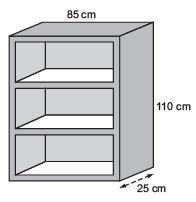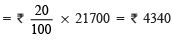Cost of painting

∵ Area to be painted = [(75 x 20) x 6] + [(90 x 20) x 2] + [90 x 75] cm
= [9000 + 3600 + 6750] cm2 = 19350 cm2
∴ Cost of painting at the rate of 10 paise per cm2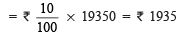Total Expenses

Total expenses = (Cost of polishing) + (Cost of painting)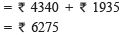Thus, the total required expense = ₹ 6275.

Question 2. The front compound wall of a house is decorated by wooden spheres of diameter 21 cm, placed on small supports as shown in Fig. Eight such spheres are used for this purpose, and are to be painted silver. Each support is a cylinder of radius 1.5 cm and height 7 cm and is to be painted black. Find the cost of paint required if silver paint costs 25 paise per cm2 and black paint costs 5 paise per cm2.
Solution:
For spherical part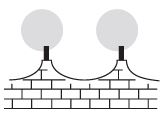Surface area of a sphere = 4pr2

∴ Surface area of a wooden sphere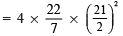[∵ Diameter = 21 cm]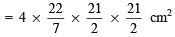= 22 x 3 x 21 cm= 1386 cm

Surface area of the tip of a pillar = π (Radius)2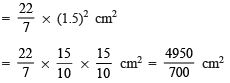= 7.071 cm2

∴ Surface area of a wooden sphere to be painted silver = [1386 – 7.071] cm
= 1378.93 cm2
⇒ Surface area of 8 wood spheres to be painted = 8 x 1378.93 cm
= 11031.44 cm
Now, the cost of silver painting at the rate of 25 paise per cm2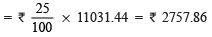For cylindrical part Radius of the base of the cylindrical part (R) = 1.5 cm
Height of the cylindrical part (h) = 7 cm
∴ Curved surface area of the cylindrical part (pillar) = 2πRh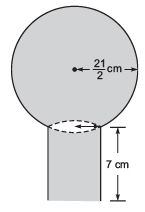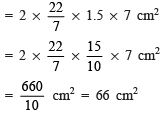⇒ Total curve surface area of 8 pillars = 8 x 66 cm2 = 528 cm2
∴ Cost of painting black for 8 pillars at the rate of 5 paise per cm2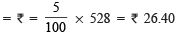Total cost of painting Total cost of painting = [Cost of silver painting] + [Cost of black painting]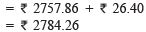Question 3. The diameter of a sphere is decreased by 25%. By what per cent does its curved surface area decrease?
Solution:
Let the original diameter = d Case I
⇒ Original surface area of the sphere =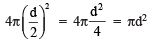Case II Decreased diameter = d – (25% of d) = d –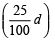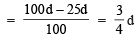∴ Decreased radius =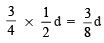⇒ Decreased surface area =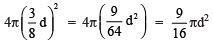Per cent decrease in surface area Decrease in curved surface area = [Original surface area] – [Decreased surface area]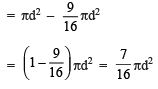Now, % decrease in surface area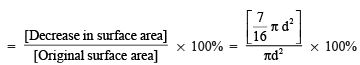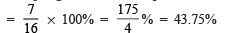Thus, the required per cent decrease in curved surface area is 43.75%

Offer running on EduRev: Apply code STAYHOME200 to get INR 200 off on our premium plan EduRev Infinity!

132 docs

,

,

,

,

,

,

,

,

,

,

,

,

,

,

,

,

,

,

,

,

,

;